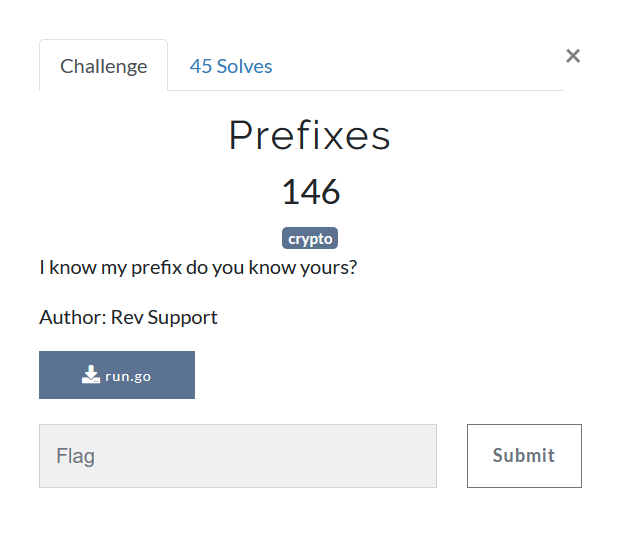# Prefixespackage main

import (
"fmt"
"strings"
)

func charCodeAt(st string, ni int) rune {
for i, j := range st {
if i == ni {
return j
}
}
return 0
}

func hexify(st string) string {
var ok string = ""
ltr := []string{"0", "1", "2", "3", "4", "5", "6", "7", "8", "9", "a", "b", "c", "d", "e", "f"}

for cnt := 0; cnt < len(st); cnt++ {
ok += ltr[charCodeAt(st, cnt)>>4] + ltr[charCodeAt(st, cnt)&15]
}

return ok
}

func encrypt(st string) string {

var initialize int = 0
var ot string = ""
var val int

for i := 0; i < len(st); i++ {
val = int(charCodeAt(st, i))
initialize ^= (val << 2) ^ val
ot += string(initialize & 0xff)
initialize >>= 8
}

return strings.Replace(hexify(ot), "00", "", -1)
}

func main() {
fmt.Println(encrypt("[REDACTED FLAG]"))
}



We are provided with this go file, and a hash which we have to reverse
TBH I dont know go, but the code is comprehendible enough in my opinion.
Lets analyse the code chunk by chunk


func charCodeAt(st string, ni int) rune {
for i, j := range st {
if i == ni {
return j
}
}
return 0
}


It should return the character value 0-255 for the character at position ni in string st

func hexify(st string) string {
var ok string = ""
ltr := []string{"0", "1", "2", "3", "4", "5", "6", "7", "8", "9", "a", "b", "c", "d", "e", "f"}

for cnt := 0; cnt < len(st); cnt++ {
ok += ltr[charCodeAt(st, cnt)>>4] + ltr[charCodeAt(st, cnt)&15]
}

return ok
}


I should hexlify, i.e. return the hexadecimal representation the the string st

func encrypt(st string) string {

var initialize int = 0
var ot string = ""
var val int

for i := 0; i < len(st); i++ {
val = int(charCodeAt(st, i))
initialize ^= (val << 2) ^ val
ot += string(initialize & 0xff)
initialize >>= 8
}

return strings.Replace(hexify(ot), "00", "", -1)
}


This should encrypt the hash obviously.
Now looking at how it does stuff, it should be easy enough to reverse all steps. We shall begin from the last step which is
strings.Replace(hexify(ot), "00", "", -1)
Which simply replaces all occurences of "00" with "" (nothing) throught (-1) from hexlified string of ot. Hence, we should be able to get ot (sans “00”) from the final hash by simply hex-decoding.

Now, since we dont know the final value of initialize, we can reverse it from the start since we know its initial value to be 0.

The character at position i is simply the val which produces initialize ^ (val<<2) ^ val with corresponding character at position i in ot

Here is a quick script to do the same

ct = "eae4a5b1aad7964ec9f1f0bff0229cf1a11b22b11bfefecc9922aaf4bff0dd3c88"
ct = bytes.fromhex(ct)
flag = ""

initialize = 0
for i in range(len(ct)):
for val in range(256):
if (initialize ^ (val<<2)^val)&0xff == ct[i]:
flag += chr(val)  #the value val is found
initialize ^= (val<<2)^val  #to get the value of initialize for nex iteration of i
initialize >>=8 # initialize is [0-3] since (val<<2)^(val) is 10 bits, which is right shifted 8 bits each iteration
break
print(flag)
#batpwn{Ch00se_y0uR_pR3fix_w1selY}


I was not expecting it to give out the flag in first go since 00 were stripped out of the final hash. Turns out this step was redundant. If it were not, we could simply use all 4 values of initialize for the places where the flag seems to not make sense.

jekyll.environment != "beta" -%}